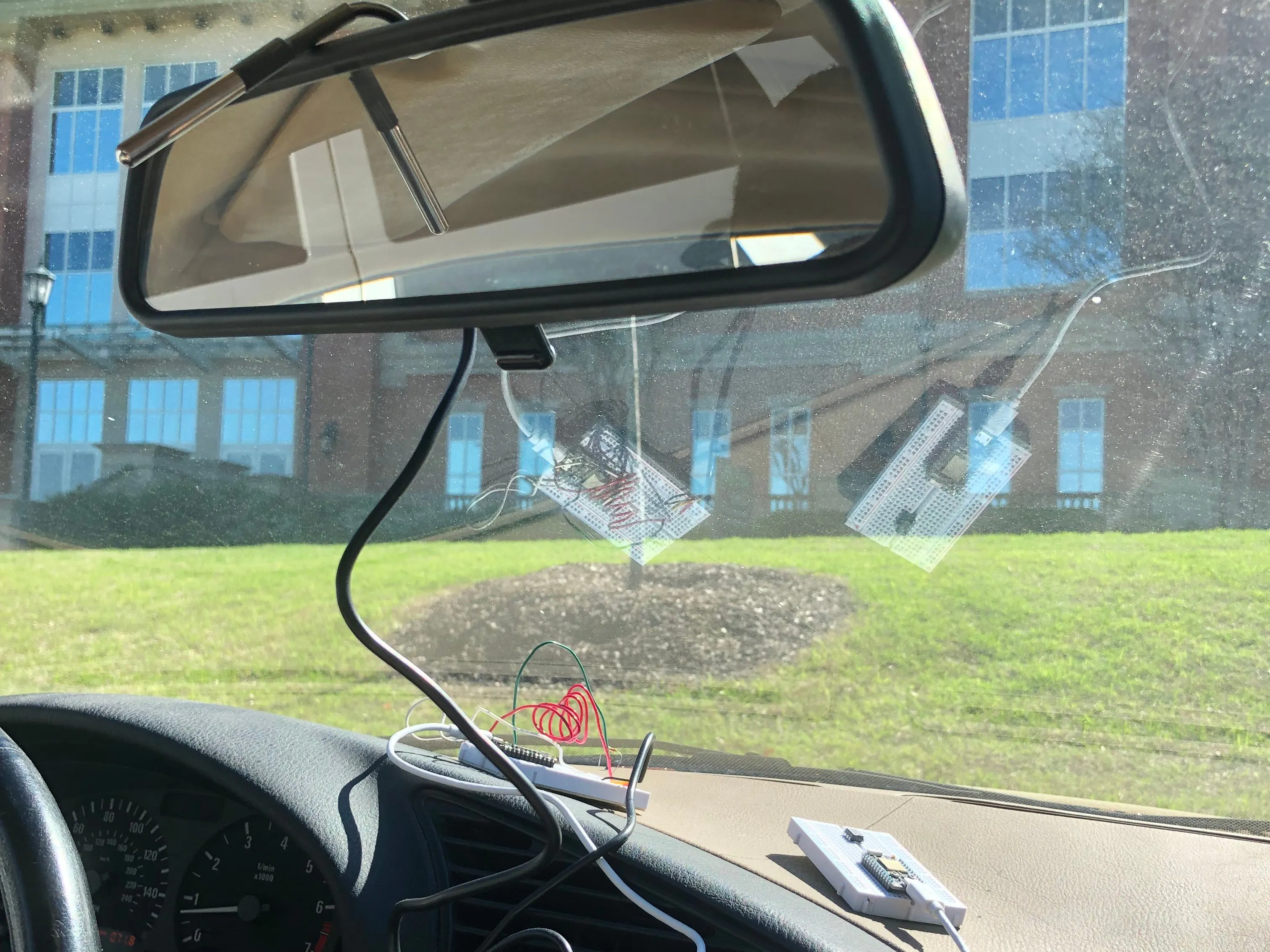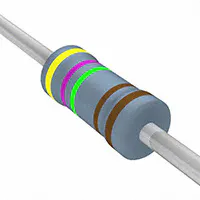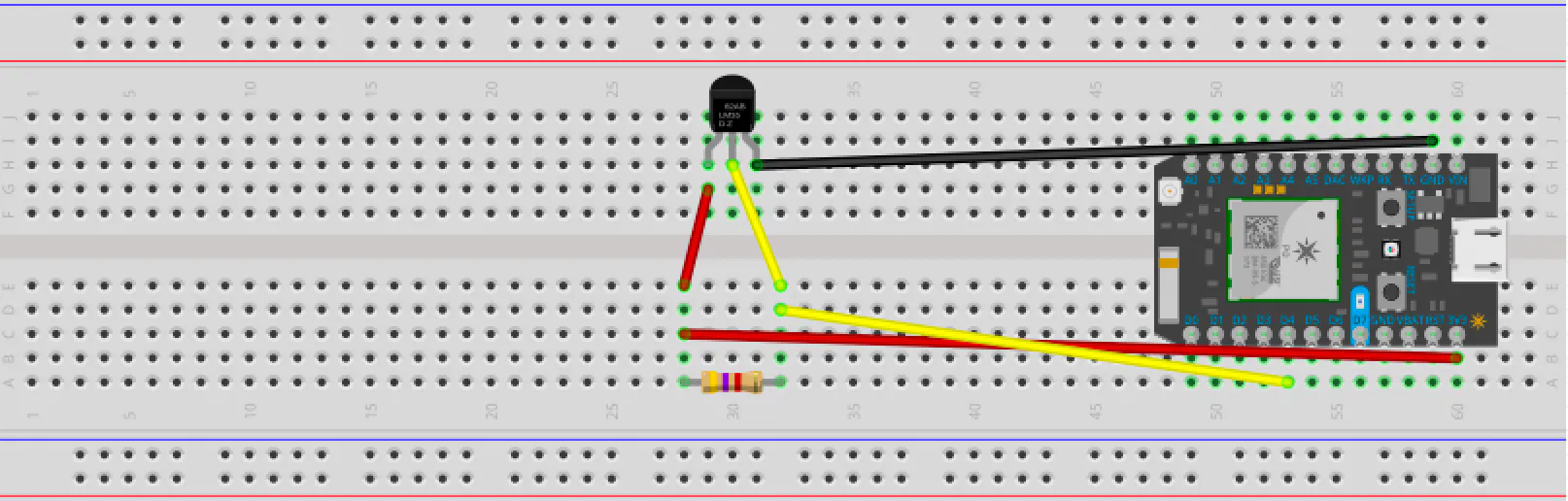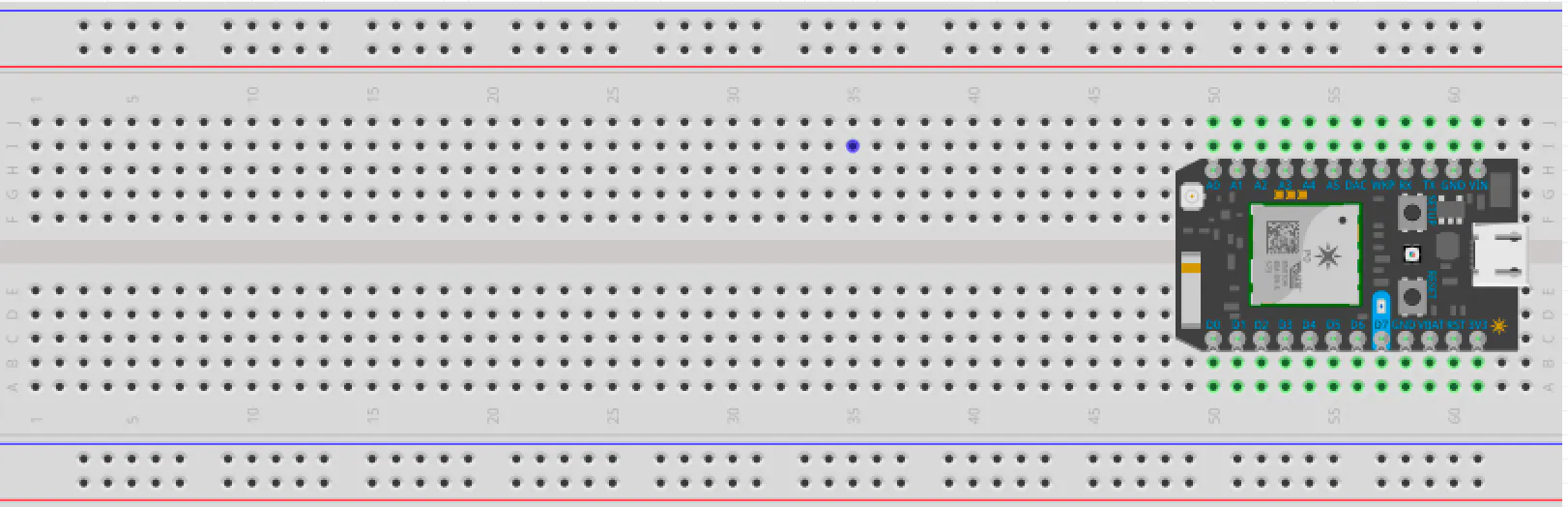Published

MEGR 3171 - That Car is HOT HOT HOT!

This project demonstrates the usage of a photon and temperature sensor programmed to notify you when your car reaches a certain temperature.

IntermediateFull instructions provided4 hours741Things used in this project

Hardware componentsParticle Photon
×2Jumper wires (generic)
×3
 Particle Temperature Sensor - (sealed)
×1Resistor 4.75k ohm
×1

Software apps and online servicesIFTTT Maker serviceParticle Build Web IDE

Schematics

Temperature Measuring Photon

Emma's PhotonThreshold Determining Photon

Dylan's PhotonCode

Threshold Determination

C/C++
This is the code for Dylan's Photon which establishes the temperature threshold and compares the data from Emma's Photon to establish if you're above or below the target temperature.
// ----------------------------------------------------------------------
// Determining Whether the Published Tmeperature has Crossed a Threshold
//-----------------------------------------------------------------------
int boardLed = D7;
int tempThreshold;
int temperature;

void setup() {
pinMode(boardLed,OUTPUT);
// Our on-board LED is output.

// Here we are going to subscribe to smol_photon's event using Particle.subscribe:
Particle.subscribe("smolphotonevent69", myHandler, "18002d001447363336383437");
// Subscribe will listen for the event smolphotonevent69 and, when it finds it, will run the function myHandler()
// myHandler() is declared later in this app.

digitalWrite(boardLed,LOW);
delay(1000);
// First, the D7 LED will be off
tempThreshold = 70;
// Initialize a threshold as the desired temperature (in degrees fahrenheit) of the car.
}

void loop() {
// This void begins a loop so ur_mom will continuously subscribe and publish.
}
// The myHandler function is called when the cloud tells us that smol_photon's event is published.
void myHandler(const char *event, const char *data)
{
digitalWrite(boardLed,HIGH);
delay(1000);
//ur_mom will blink for a second each time smol_photon publishes a new temperature

int myValue = atoi(data);
//Convert the string received from the smolphotonevent69 into an integer.
if (myValue>tempThreshold) {
// Compare the incoming data with the chosen threshold.
Particle.publish("bigphotonevent420","above");
// Publish a public event announcing the temperature to be above the threshold.
digitalWrite(boardLed,LOW);
delay(2000);
// If the temp is above the threshold, then the LED will keep blinking.
}

else {
Particle.publish("smolphotonevent420","below");
// Publish a public event announcing the temperature to be below the threshold.
digitalWrite(boardLed,HIGH);
delay(2000);
// If the temp is below the threshold, the LED will stay on.
}
}

Temperature Sensor

C/C++
This is the code on Emma's Photon for temperature measurement, slightly modified from the Particle Tutorial mentioned above.
// This #include statement was automatically added by the Particle IDE.
#include <OneWire.h>

/************************************************************************
This sketch reads the temperature from a 1-Wire device and then publishes
to the Particle cloud. From there, IFTTT can be used to log the date,
here: https://docs.particle.io/tutorials/topics/maker-kit

This sketch is the same as the example from the OneWire library, but
with the addition of three lines at the end to publish the data to the
cloud.

Use this sketch to read the temperature from 1-Wire devices
you have attached to your Particle device (core, p0, p1, photon, electron)

Temperature is read from: DS18S20, DS18B20, DS1822, DS2438

Expanding on the enumeration process in the address scanner, this example
reads the temperature and outputs it from known device types as it scans.

I/O setup:
These made it easy to just 'plug in' my 18B20 (note that a bare TO-92
sensor may read higher than it should if it's right next to the Photon)

D3 - 1-wire ground, or just use regular pin and comment out below.
D4 - 1-wire signal, 2K-10K resistor to D5 (3v3)
D5 - 1-wire power, ditto ground comment.

A pull-up resistor is required on the signal line. The spec calls for a 4.7K.
I have used 1K-10K depending on the bus configuration and what I had out on the
bench. If you are powering the device, they all work. If you are using parisidic
power it gets more picky about the value.
************************************************************************/

OneWire ds = OneWire(D4);  // 1-wire signal on pin D4

unsigned long lastUpdate = 0;
int led = D7;
float lastTemp;
void setup() {
Particle.subscribe("smolphotonevent420",anything,"430035000e47363336383437");
pinMode(led,OUTPUT);
digitalWrite(led,LOW);
Serial.begin(9600);

}
void anything(const char *event, const char *data)
{

digitalWrite(led,HIGH);
delay(2500);
digitalWrite(led,LOW);
//if temp is below threshold, smol_photon will blink

}

// up to here, it is the same as the address acanner
// we need a few more variables for this example

void loop(void) {
byte i;
byte present = 0;
byte type_s;
byte data;
float celsius, fahrenheit;

Serial.println();
ds.reset_search();
delay(250);
return;
}

// The order is changed a bit in this example
// first the returned address is printed

Serial.print("ROM =");
for( i = 0; i < 8; i++) {
Serial.write(' ');
}

// second the CRC is checked, on fail,

Serial.println("CRC is not valid!");
return;
}
Serial.println();

// we have a good address at this point
// what kind of chip do we have?
// we will set a type_s value for known types or just return

// the first ROM byte indicates which chip
case 0x10:
Serial.println("  Chip = DS1820/DS18S20");
type_s = 1;
break;
case 0x28:
Serial.println("  Chip = DS18B20");
type_s = 0;
break;
case 0x22:
Serial.println("  Chip = DS1822");
type_s = 0;
break;
case 0x26:
Serial.println("  Chip = DS2438");
type_s = 2;
break;
default:
Serial.println("Unknown device type.");
return;
}

// this device has temp so let's read it

ds.reset();               // first clear the 1-wire bus
ds.select(addr);          // now select the device we just found
// ds.write(0x44, 1);     // tell it to start a conversion, with parasite power on at the end
ds.write(0x44, 0);        // or start conversion in powered mode (bus finishes low)

// just wait a second while the conversion takes place
// different chips have different conversion times, check the specs, 1 sec is worse case + 250ms
// you could also communicate with other devices if you like but you would need

delay(1000);     // maybe 750ms is enough, maybe not, wait 1 sec for conversion

// we might do a ds.depower() (parasite) here, but the reset will take care of it.

// first make sure current values are in the scratch pad

present = ds.reset();
ds.write(0xB8,0);         // Recall Memory 0
ds.write(0x00,0);         // Recall Memory 0

present = ds.reset();
if (type_s == 2) {
ds.write(0x00,0);       // The DS2438 needs a page# to read
}

// transfer and print the values

Serial.print("  Data = ");
Serial.print(present, HEX);
Serial.print(" ");
for ( i = 0; i < 9; i++) {           // we need 9 bytes
Serial.print(data[i], HEX);
Serial.print(" ");
}
Serial.print(" CRC=");
Serial.print(OneWire::crc8(data, 8), HEX);
Serial.println();

// Convert the data to actual temperature
// because the result is a 16 bit signed integer, it should
// be stored to an "int16_t" type, which is always 16 bits
// even when compiled on a 32 bit processor.
int16_t raw = (data << 8) | data;
if (type_s == 2) raw = (data << 8) | data;
byte cfg = (data & 0x60);

switch (type_s) {
case 1:
raw = raw << 3; // 9 bit resolution default
if (data == 0x10) {
// "count remain" gives full 12 bit resolution
raw = (raw & 0xFFF0) + 12 - data;
}
celsius = (float)raw * 0.0625;
break;
case 0:
// at lower res, the low bits are undefined, so let's zero them
if (cfg == 0x00) raw = raw & ~7;  // 9 bit resolution, 93.75 ms
if (cfg == 0x20) raw = raw & ~3; // 10 bit res, 187.5 ms
if (cfg == 0x40) raw = raw & ~1; // 11 bit res, 375 ms
// default is 12 bit resolution, 750 ms conversion time
celsius = (float)raw * 0.0625;
break;

case 2:
data = (data >> 3) & 0x1f;
if (data > 127) {
celsius = (float)data - ((float)data * .03125);
}else{
celsius = (float)data + ((float)data * .03125);
}
}

// remove random errors
if((((celsius <= 0 && celsius > -1) && lastTemp > 5)) || celsius > 125) {
celsius = lastTemp;
}

fahrenheit = celsius * 1.8 + 32.0;
lastTemp = celsius;
Serial.print("  Temperature = ");
Serial.print(celsius);
Serial.print(" Celsius, ");
Serial.print(fahrenheit);
Serial.println(" Fahrenheit");
// now that we have the readings, we can publish them to the cloud
String temperature = String(fahrenheit); // store temp in "temperature" string
Particle.publish("smolphotonevent69", temperature);// publish to cloud
delay(5000);} // 5 second delay

Credits

Emma Nichols

1 project • 1 follower